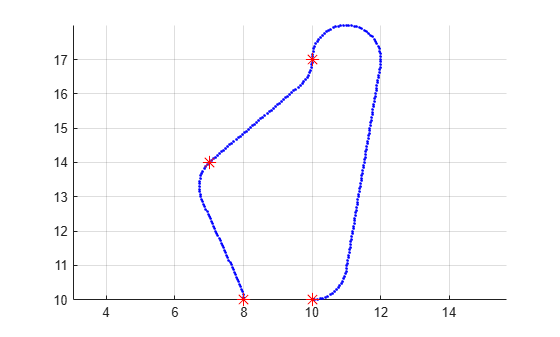navPath

Planned path

Description

The navPath object stores paths that are typically created by geometric path planners. Path points are stored as states in an associated state space.

Creation

Description

path = navPath creates a path object, path, using the SE2 state space with default settings.

example

path = navPath(space) creates a path object with state space specified by space. The space input also sets the value of the StateSpace property.

path = navPath(space,states) allows you to initialize the path with state samples given by states. Specify states as a matrix of state samples. States that are outside of the StateBounds of the state space object are reduced to the bounds. The states input also sets the value of the States property.

Properties

expand all

State space for the path, specified as a state space object. Each state in the path is a sample from the specified state space. You can use objects such as stateSpaceSE2, stateSpaceDubins, or stateSpaceReedsShepp as a state space object. You can also customize a state space object using the nav.StateSpace object.

Data Types: object

States of the path, specified as a real-valued M-by-N matrix. M is the number of states in the path, and N is the dimension of each state. You can only set this property during object creation or using the append function.

Data Types: double

Number of state samples in the path, specified as a nonnegative integer. The number is the same as the number of rows of the state matrix specified in the States property.

Data Types: double

Object Functions

 append Add states to end of path copy Create copy of path object interpolate Interpolate points along path pathLength Length of path

Examples

collapse all

Create a navPath object based on multiple waypoints in a Dubins space.

dubinsSpace = stateSpaceDubins([0 25; 0 25; -pi pi])
dubinsSpace =
stateSpaceDubins with properties:

SE2 Properties
Name: 'SE2 Dubins'
StateBounds: [3×2 double]
NumStateVariables: 3

Dubins Vehicle Properties

pathobj = navPath(dubinsSpace)
pathobj =
navPath with properties:

StateSpace: [1×1 stateSpaceDubins]
States: [0×3 double]
NumStates: 0

waypoints = [...
8 10 pi/2;
10 12 pi/4;
12 17 pi/2;
11 10 -pi];
append(pathobj, waypoints);

Interpolate that path so that it contains exactly 250 points.

interpolate(pathobj, 250)

Visualize the interpolated path and the original waypoints.

figure;
grid on;
axis equal;
hold on;
plot(pathobj.States(:,1), pathobj.States(:,2), ".b");
plot(waypoints(:,1), waypoints(:,2), "*r", "MarkerSize", 10)Calculate length of path.

len = pathLength(pathobj);
disp("Path length = " + num2str(len))
Path length = 19.37